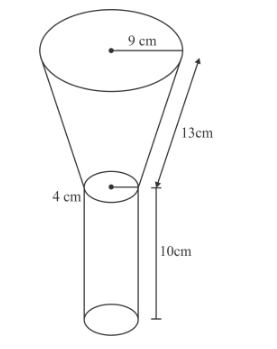# An oil funnel of tin sheet consists of a cylindrical portion 10 cm`
Question:

An oil funnel of tin sheet consists of a cylindrical portion 10 cm long attached to a frustum of a cone. If the total height be 22 cm, the diameter of the cylindrical portion 8 cm and the diameter of the top of the funnel 18 cm, find the area of the tin required.

Solution:Let r1 and r2 (r1 > r2) be the radii of the ends of the frustum of a cone. Suppose l and h be the slant height and height of frustum of cone.

Diameter of top of the frustum $2 r_{1}=18 \mathrm{~cm}$

$r_{1}=9 \mathrm{~cm}$

Diameter of bottom of frustum $2 r_{2}=8 \mathrm{~cm}$

$r_{2}=4 \mathrm{~cm}$

Let h1 be the height of the cylindrical portion.

Now, h1 = 10 cm

Total height of the funnel = 22 cm

height of frustum of cone, h = 22−10 = 12 cm

$l=\sqrt{\left(r_{1}-r_{2}\right)^{2}+h^{2}}$

$\Rightarrow l=\sqrt{(9-4)^{2}+(12)^{2}}$

$\Rightarrow l=\sqrt{25+144}$

$\Rightarrow l=\sqrt{169}$

$\Rightarrow l=13 \mathrm{~cm}$

Curved surface area of funnel = CSA of frustum of cone + CSA of cylinder

Curved surface area of funnel $=\pi\left(r_{1}+r_{2}\right) l+2 \pi r_{2} h_{1}$

Therefore,

Area of the tin required

$=\pi\left(r_{1}+r_{2}\right) l+2 \pi r_{2} h_{1}$

$=\pi\left[\left(r_{1}+r_{2}\right) l+2 r_{2} h_{1}\right]$

$=\pi[(9+4) \times 13+2 \times 4 \times 10]$

$=\pi[169+80]$

$=\pi$

$=249 \pi \mathrm{cm}^{2}$